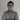# You have to love JavaScript’s type coercion

June 28th, 2014 | 5 min read | coercion, JavaScript

JavaScript… some people love it, other people hate it. One of the many good/bad discussions in JavaScript are about its weak and dynamic typing. Because of that, type coercion exists. Type coercion you ask? Well, type coercion is a feature in JavaScript that automatically “casts” one of the operands to another type, for example:

``[] == "" // true``

This can result in some really funny things, for example:

``````[] == 0; // true
0 == "0"; // true``````

So, as you can see here, an empty array equals zero and it also equals the string literal `"0"`. So, a mathematical conclusion would be that the following returns `true` as well:

``[] == "0"; // What does it return?``

However, if you execute it, you will notice that this is not the case, while `[]` is equal to 0 and 0 is equal to `"0"`, `[]` is not equal to `"0"`.

If you then combine some boolean operations with it, you can get some really weird stuff, for example:

``![] == []; // true``

This feature can become really handy to check certain things, but it can also be a real pain if you don’t know what’s going on there.

In this “fun with JavaScript” tutorial I will try to write my name (which is “Dimitri”), without using any character or String literal, so let’s start.

### Letter ‘d’

To get the letter ‘d’, the easiest thing to do is get a string containing ‘undefined’. In this case I’m going to try to get `undefined` by retrieving a non existing array element, for example:

``[][[]]; // undefined``

What it tries to do here is trying to get the element `[]` from an empty array, which is obviously not existing.

You can do some really funny stuff with associative arrays in JavaScript, for example:

``````var a = []; // New array
a[[]] = "test";
a[[]]; // returns "test"
a[""]; // returns "test"``````

So as you can see, the type coercion casts an empty array as argument to an empty string, as the element can be retrieved by both operations.

Back to the letter ‘d’, we now have `undefined` but have to convert it to a string now. As I just told, an empty array can be casted to a string, so if you add a keyword like `undefine` to a string, it becomes a string, for example:

``[]+[][[]]; // "undefined"``

A string can also be seen as an array, where each index of the array contains a single letter. So, in this case the letter ‘d’ is the element at index 2 (because it’s zero based).

To get the number 2, we can do some boolean arithmetic operations, for example:

``````!![]; // true
!![]+!![]; // 2``````

Everything combined we get:

``([]+[][[]])[!![]+!![]]; // "d"``

That was fun, isn’t it?

### The letter ‘i’

The letter ‘i’ is quite simple now, because that’s the character at the 5th index of `"undefined"`.

So, we now get something like:

``([]+[][[]])[!![]+!![]+!![]+!![]+!![]]; // "i"``

### Halfway with “m”

The letter “m” is a bit more difficult. The easiest way to get it is by using `typeof` on a numeric value, which would return the string `"number"`. We already know how to get a number, for example:

``````!![]+!![]; // 2
![]+![]; // 0``````

So, to get the type of it we can use:

``typeof (![]+![]); // "number"``

Now we have to get the character at index 2 to retrieve the letter “m”:

``(typeof (![]+![]))[!![]+!![]]; // "m"``

### AlmosT There (letter “t”)

We’re almost there now (luckily I have many “i”‘s in my name). To get the letter “t” we can try to get the string `"true`“.

If you remember things well, you know that you can get a boolean `true` by using:

``!![]; // true``

So, to make it into a string, we concatenate it with an empty array like we did with the string `"undefined"`:

``[]+!![]; // "true"``

Finally, we just have to get the character at index 0, which we know how to do now:

``([]+!![])[![]+![]]; // returns "t"``

### Easy peasy “r”

The letter “r” is not that hard now, like the “t”, we can get it from the string `"true`“, the only thing we have to change is the index:

``([]+!![])[![]+!![]]; // "r"``

### All together now

So now we made it possible to get each letter of my name, now we only have to concatenate them all together and it becomes:

``([]+[][[]])[!![]+!![]]+([]+[][[]])[!![]+!![]+!![]+!![]+!![]]+(typeof (![]+![]))[!![]+!![]]+([]+[][[]])[!![]+!![]+!![]+!![]+!![]]+([]+!![])[![]+![]]+([]+!![])[![]+!![]]+([]+[][[]])[!![]+!![]+!![]+!![]+!![]]; // "dimitri"``

Or test it out by yourself by pasting the following at your address bar:

``javascript:alert(([]+[][[]])[!![]+!![]]+([]+[][[]])[!![]+!![]+!![]+!![]+!![]]+(typeof (![]+![]))[!![]+!![]]+([]+[][[]])[!![]+!![]+!![]+!![]+!![]]+([]+!![])[![]+![]]+([]+!![])[![]+!![]]+([]+[][[]])[!![]+!![]+!![]+!![]+!![]])``
Note: Most browsers filter the `javascript:` part out of the string when copy pasting it in your address bar due to safety reasons.
So, make sure to put it back there in case it was filtered.

So, from now on, if you’re bored you know what to do 😉